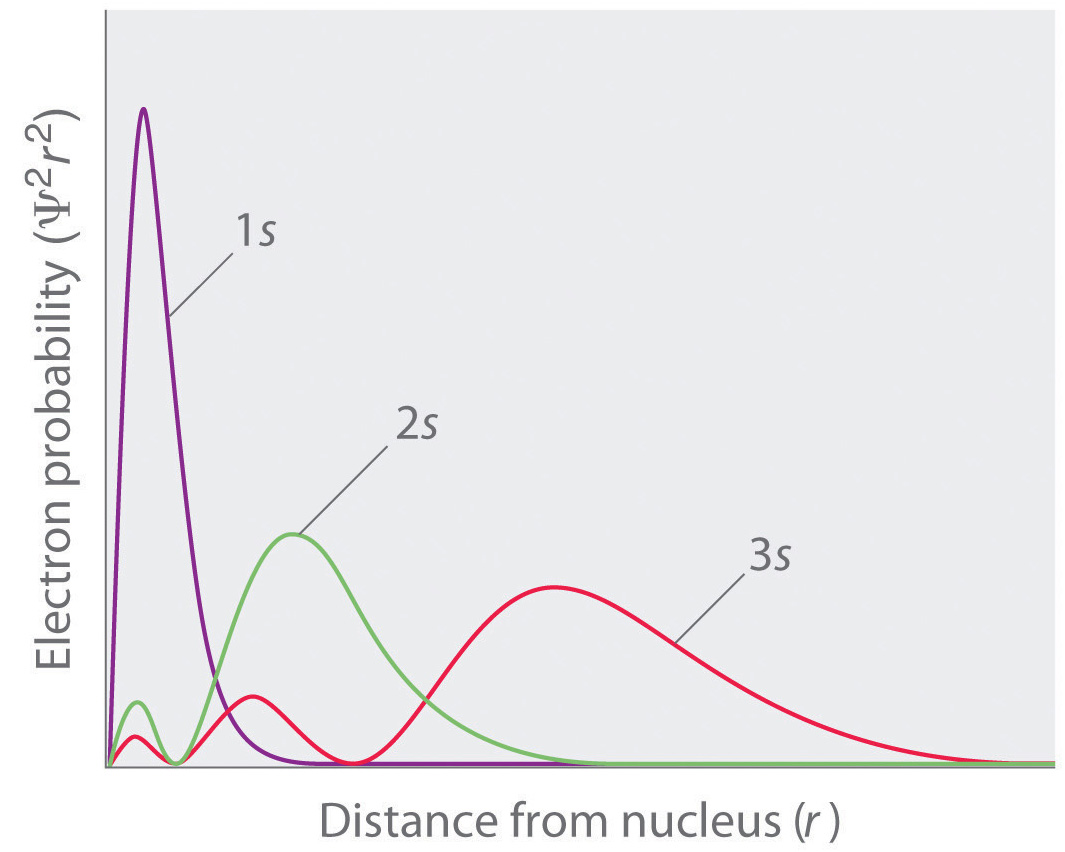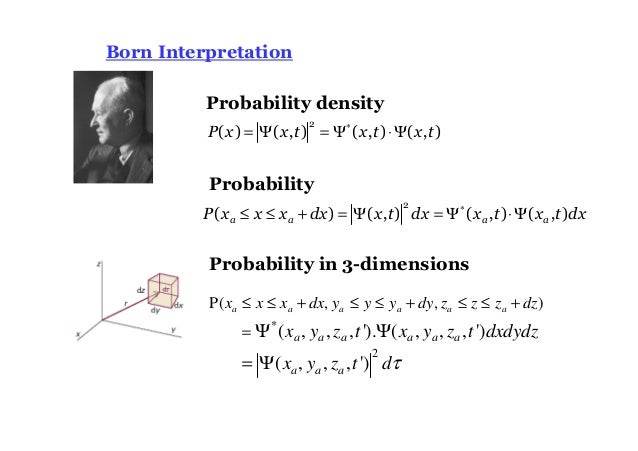## News

• ElkArte - Just Installed!

##Topic: Schrodinger's Equation and Basic Quantum Mechanics Refresher (Read 2967 times)previous topic - next topic

• stcordova
•• Global Moderator
Schrodinger's Equation and Basic Quantum Mechanics Refresher
##### February 02, 2018, 04:46:59 PM
This thread is my personal "notebook" to re-teach myself Schrodinger's equations and their implications.  Amazingly, Tipler and Barrow generalized Schrodinger's equation to argue God exists, but I shall start out more modestly.  :-)

• stcordova
•• Global Moderator
Re: Schrodinger's Equation and Basic Quantum Mechanics Refresher
##### Reply #1 – February 02, 2018, 05:03:58 PM
This thread is a spin-off of the discussion here:

http://theskepticalforum.org/index.php?topic=347.0

As alluded to in that thread the Bohr model of the electron in a hydrogen atom describes the AVERAGE behavior of an ensemble of hypothetical electrons based on the energy state of the electron. The change in energy associated with "orbits" of the electron is described by

E = h * nu

But if we have average behavior, what the is the equation that describes the probability distribution from which we get averages from.  For example, here is one distribution with an average, the normal distribution.  Graphically depicted the distribution looks like:In terms of an equation, it is described exactly as:Unfortunately, the distribution of positions of a quantum "particle" like an electron isn't so easy to depict as it is dependent on context.

But let's look at a specific example,  the electron "orbiting" the hydrogen atom.  The average position accords with the bohr model.  If we plotted a graphical distribution of the distance of the electron from the center of the nucleus of the hydrogen atom, the probability distribution for the various "orbits" would look like:That is to say, if we had a machine that recorded positions of the electron in a partical energy state (like 1s) over time, and plotted how many times the electron appeared at various distances, it would look like the plot above.

To mathematically capture this, Shrodinger had to make an equation to predict that behavior and hence the famous Shrodinger equation:• stcordova
•• Global Moderator
Re: Schrodinger's Equation and Basic Quantum Mechanics Refresher
##### Reply #2 – February 02, 2018, 05:12:59 PM
Now the Schrodinger Equation looks intimidating, but really it's just an equation with parameters.  The most important are:

m = mass
V= potential energy
h-bar = planck's constant/ 2 pi

A mathematician can then take those parameters and solve the equation of Psi (the pitchfork symbol).  Hypothetically, someone could write a computer program, and I could just pump in the parameters, and I get solutions to Psi.

It turns out one can then derive the probability distributions based on Psi by this equation by Max Born:Max Born won the Nobel Prize for his work on Quantum Mechanics.

As a total aside, Max Born was the grand father of this famous singer and actress, Olivia Newton John:• stcordova
•• Global Moderator
Re: Schrodinger's Equation and Basic Quantum Mechanics Refresher
##### Reply #3 – February 02, 2018, 05:18:23 PM
Well the Nobel Prize winning Quantum Physicist Max Born produced some headaches for physics students but he produced a talented grand daughter to sooth some of those headaches.  Here is Born's grand daughter, Olivia Newton John singing and making God's world a friendlier place to live:

"I honestly love you" performed by a Quantum Physicists Grand Daughter

• stcordova
•• Global Moderator
Re: Schrodinger's Equation and Basic Quantum Mechanics Refresher
##### Reply #4 – February 02, 2018, 06:07:03 PM
Trying to learn solutions to Shrodinger's equation as applied to real world systems is very difficult, and in fact for even simple systems, virtually impossible.  So we generally start with trivial hypothetical toy systems.

Schrodinger's equation isn't actually a solution, it describes what the solution to the equation must be, and the solution is called Psi (the pitchfork symbol).

Stepping back a bit outside of Schrodinger's equation, lets look at a trivial differential equation where the solution is "Y", not "Psi"Now, notice the differential equation has an infinite number of possible solutions. In other words, there are an infinite number of possible Ys that can satisfy the differential equation.  Now, just for fun, I can substitute Psi in place of Y in the differential equation and it's derivation of a solution.  It's all just symbol manipulation. I'm doing this to try to de-mystify what we are aiming to do with the Schrodinger differential equation by putting Psi in a trivial differential equation:

So that was the Psi solution to a trivial differential equation.  In Quantum Mechanics in many non-relativistic contexts for the simplest case, the Psi solution is a solution to this 2nd order partial equation in two dimenstions, namely Shrodinger's equation for a simple system: